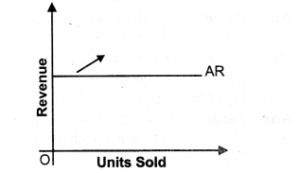# Chapter 10 – Perfect Competition Questions and Answers: NCERT Solutions for Class 12 Micro Economics

Class 12 Introduction to Economics NCERT book solutions for Chapter 10 - Perfect Competition Questions and Answers.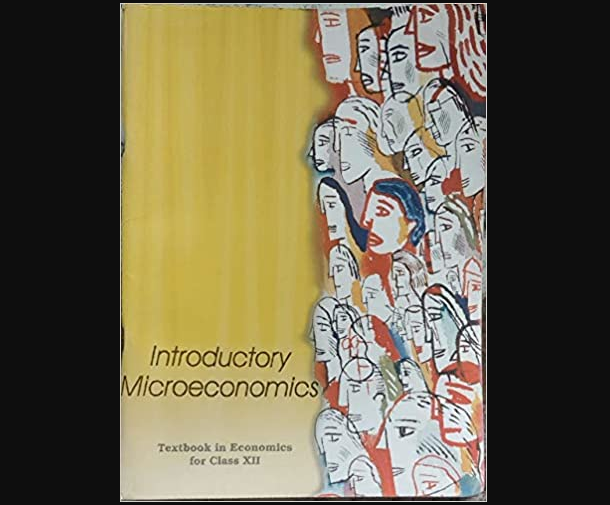## Question 2. What is price line under perfect competition?

### Answer : 1. The price line shows the relationship between the market price and a competitive firm’s output level, 2. The vertical height of the price line is equal to the market price as shown in the given figure.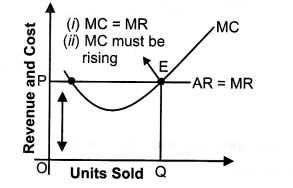## Question 2. “In perfect competition, industry is the price maker and firm is the price taker.” Discuss.

### Answer: Yes, this statement is true. 1. As we know, in Perfect competition, homogeneous goods are produced. So, industry cannot charge different price from different firms. 2. So, industry will give that price to the firm where industry is in equilibrium, i.e., where Demand = Supply. Any movement from that point would be unstable.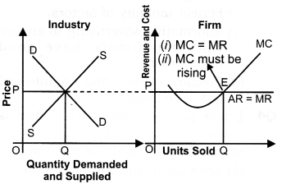## Question 4. What is the relationship between TR, AR and MR under perfect competition?

### Answer: 1. In the perfect competition, a firm is a price taker. 2. It has to sell its product at the same price as given (determined) by the industry. Consequently, price = AR = MR. 3. Hence, a firm’s AR and MR curve will be a horizontal straight line parallel to X axis. 4. Since price remains the same, i.e., MR is constant, therefore, TR increases at the Constant rate as increase in the output sold. 5. As the result of, TR curve facing a competitive firm is positively sloped straight line. Again, because at zero output Total Revenue is zero therefore, TR curve passes through the origin O as shown in the given figure.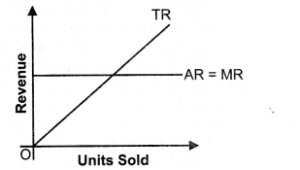## Question 5. Why is AR curve of a firm under perfect competition parallel to X-axis?

### Answer: 1. AR curve of a firm under perfect competition is parallel to X-axis because in perfect competition homogeneous product are produced, that is why price remains constant and as we know AR = TR/Q = PX Q/Q = Price. So, AR remains constant. 2. As, AR is on Y-axis, that is why AR curve remains constant and parallel to X-axis.